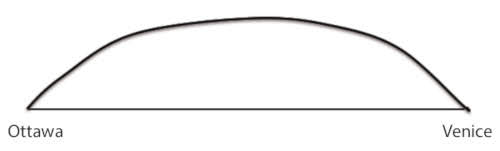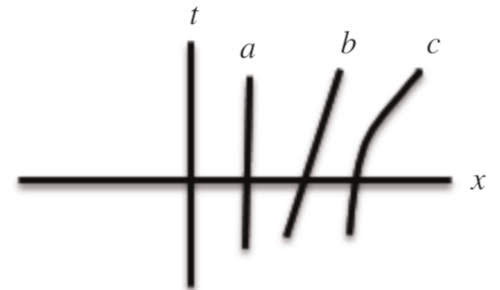### Spacetime analogies

The elastic sheet model is one of the most used model for telling general relativity. It allows to show as smaller balls "orbit" around the larger ball in the center of the sheet in a way similar to the planets, at least until the balls lose energy due to friction and finally fall into the "gravitational wall".
This way to see the general relativity is directly connected with the embedded diagrams and Flamm paraboloids, the mathematical way to see the spacetime deformations. But this analogy has some problems not only because is inaccurate like all analogies, but also becuase it could be confusing about distorced space and spacetime especially among students. So we can ask: why is the sheet deformed? Because of the weight? This fact implies the use of a circluar argument: usinf gravity to explain gravity! But if the ball isn't in spacetime, where is it?(1)
Despite this objections, I must note that the previous questions have never been exposed in the few activities with the elastic sheet I made, probably because I ever try to explain that the elastic sheet is only an analogy needed to see some particular aspects about gravity. In every case I think that it could be very interesting to use an alternative method to tell spacetime deformation.Suppose we want to go from Ottawa to Venice by plane. This, which moves relatively close to the planet's surface, will make an arc of circumference to go from the canadian town to the italian one, instead of taking the straight line that connects the two cities directly. To see how, in the presence of gravity, this curve is the most suitable one, we can show three distinct kinds of relativistic curves on the spacetime plane.In this kind of diagrams, line $a$ is a particle at rest, the line $b$ is a particle moving with constant speed, the line $c$ is a particle moving with constant acceleration. Therefore the arc of circumference between Ottawa and Venice is the curve of an accelerated motion with acceleration equal to \$g£, in the case of the Earth(1).
Further reading: Deviation of Light near the Sun in General Relativity by Christian Magnan: original version, and archived version.
1. Janis, A. I. (2018). On mass, spacetime curvature, and gravity. The Physics Teacher, 56(1), 12-13. doi:10.1119/1.5018679 (4shared